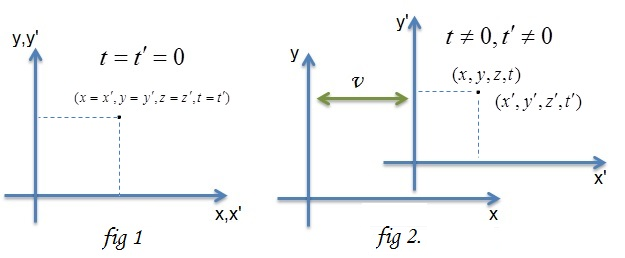## Pages

### S8. Derivaiton of Lorentz transformation Equation

In the diagram below, suppose there are two reference frames (systems) designated by $S$ and ${S}'$ such that the co-ordinate axes are parallel (as in figure). In S, we have the co-ordinates $(x,y,z,t)$ and in ${S}'$ we have the co-ordinates $({x}', {y}',{z}',{t}')$. ${S}'$ is moving with respect to $S$ with velocity $v$ (as measured in $S$) in the $x$ direction. The planes $y=0$ and $z=0$ always coincide with the planes ${y}’=0$ and ${z}’=0$, and we can set the zero points on the clocks so that $t={t}’=0$ at the instant when $x={x}’$. Although, $z$ and ${z}'$ axis is not shown in the figure one has to think it as an axis perpendicular to the page. And also a reminder here that $fig 1$ and $fig 2$ are the two instances of the reference frame $S$ and ${S}'$.Now imagine a light travelling in the positive $x$ direction in $S$ and ${S}'$. Then, a light-signal, which is proceeding along the positive axis of $+x$ in $S$ frame, is transmitted according to the equation,
$x-ct=0$

Similarly, in ${S}'$ frame, the light signal is transmitted according to the equation,
${x}'-c{t}'=0$

Remember here that we have made use of the second postulate of the special theory of relativity, i.e. “speed of light is constant in these two inertial frame..”

Those space-time points (events) which satisfy in $S$ frame must also satisfy in ${S}'$ frame, this will be the case when the following relation hold,
${x}'-c{t}'$= $\lambda$$(x-ct) (1) where, \lambda is a constant. It is clear that when (x-ct) disapears, {x}'-c{t}' disappears too. Similarly, for the light travelling which is proceeding along the negative axis of -x gives us the following relation, {x}'+c{t}'= \mu$$(x+ct)$                                               $(2)$

By adding (or subtracting) equations $(1)$ and $(2)$, we have;

and introducing for convenience the constants $a$ and $b$ in place of $\mu$'s and $\lambda$'s,  we can rewrite the equation above as,

${x}'$= $ax$$- bct (3) c{t}'= act$$- bx$                                                      $(4)$

It is clear that this is the required transformation if we know the values of the contants $a$ and $b$.

Now, in the ${S}'$ frame the value of the origin is always ${x}'=0$, putting the value in the equation $(3)$ we have,
$(x/t)=(bc/a)=v$                                                $(5)$

Since, the origin of the ${S}'$ frame is moving at constant velocity $v$ relative to the $S$ frame.

Now if we re-arrange equation $(4)$ we have the following,

${t}'$= $a[t$$-( b/ac)x] (6) then from (5), equation (6) becomes, {t}'= a[t$$-( v/c^{2})x]$                                               $(7)$

We have eliminated the constant $b$ from the equation, as can be seen in equation $7$. For, constant $a$ we proceed the following logic. At instant when $t=0$ we have from equation $3$, the following relation between the measurement of length in two different frame,

${x}'$= $ax$                                                                $(8)$

Now, at some other instances when the ${S}'$ frame is moving with velocity $v$ and the observer takes the measurement,  he should still observe the same length. So, when we take a snap shot when ${t}'=0$ we find that $(6)$ reduces to,

${t}$= $( v/c^{2})x$                                                     $(9)$

eliminating, $t$ from equation $(3)$ we have,

${x}'$$a[1- v^{2}/c^{2}]x (10) Now, the important part but tricky one is as follows, the principle of relativity teaches us that, as judged from S, the length of a unit measuring-rod which is at rest with reference to {S}' must be exactly the same as the length, as judged from {S}', of a unit measuring-rod which is at rest relative to S We thus have that, taking one of the length as the unit length an equating them we have as follows, \Delta x= 1/a \Delta {x}'$$a[1- v^{2}/c^{2}]$
Hence,

Substituting the value of $a$ in $(3)$ and $(4)$ we get the final form of the equation, the Lorentz Transformation

Thus, we have just derived the Lorentz Transformation equations.

In the next notes, we will analyse the  Lorentz Transformation equation in detail. In particular, the concept of time dilation and the length contractions.